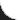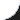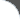Home | Tutorials | Articles | Videos | Products | Tools | Search
 Interviews | Open Source | Tag Cloud | Follow Us | Bookmark | Contact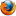In Browser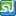StumbleUpon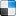del.icio.usGoogle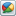Google Buzz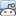redditLinkedInFacebook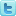TwitterLinkedinE-Mail
 Tools and Libs > Uncommons Maths > Factorial

# Factorial

Uncommons Maths is a java library with nice math utilities and random number generators. This requires the library uncommons-maths-1.2.2.jar to be in classpath. The following example shows using Maths.factorial() API. It lets us to calculate the factorial in the range 0 - 20.

 File Name  : com/bethecoder/tutorials/uncommons/FactorialTest.java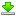Author  : Sudhakar KV Email  : [email protected]

 ``` package com.bethecoder.tutorials.uncommons; import org.uncommons.maths.Maths; public class FactorialTest {   /**    * @param args    */   public static void main(String[] args) {          int num = 5;     long factorial = Maths.factorial(num);     System.out.println("Factorial of " + num + " is " + factorial);          num = 10;     factorial = Maths.factorial(num);     System.out.println("Factorial of " + num + " is " + factorial);          num = 15;     factorial = Maths.factorial(num);     System.out.println("Factorial of " + num + " is " + factorial);   } }```

It gives the following output,
```Factorial of 5 is 120
Factorial of 10 is 3628800
Factorial of 15 is 1307674368000
```